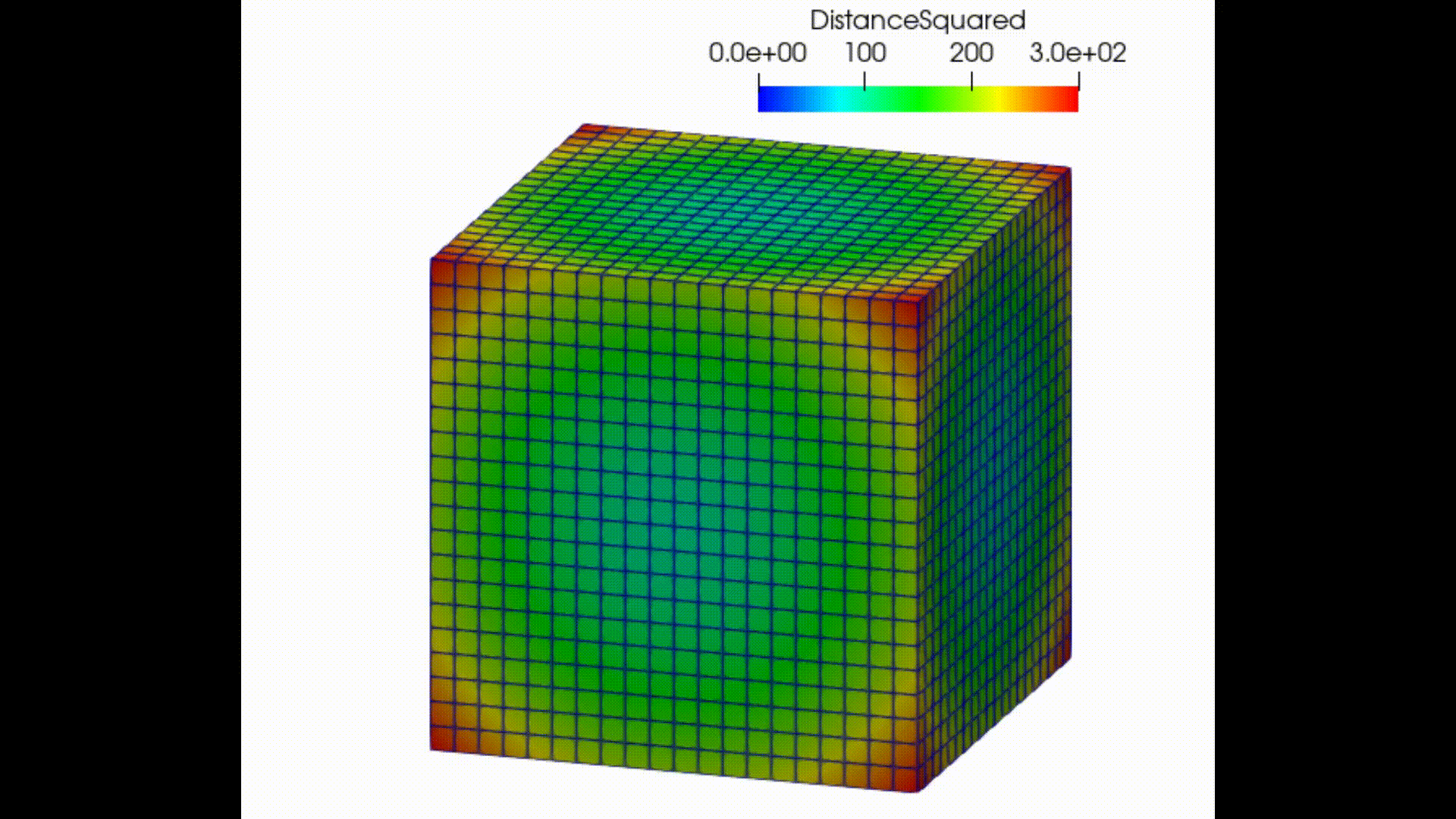# Using Point Data as Cell Centers in the Surface With Edges Visualisation

Dear community,

I would like to use ParaView to inspect the results I obtain from a cell-centered solver. The results obtained from the solver correspond to the midpoints of cubic volumes. However, ParaView interprets them as the vertex values of a structured grid. When choosing “Surface With Edges” as visualization this becomes obvious. In that case, I obtain a volume with 21 cubic cells in each direction instead of 22. I have visualized my problem in the enclosed figure. The dark points are the raw data that are interpreted as vertice values of a structured grid. The pink values would be the vertices of the larger grid, in which the raw data corresponds to the cell centers.

I am looking for a way to tell ParaView that the source data are the cell midpoints instead of the cell vertices. Is there any straightforward way to do this? I guess it requires extrapolation from the cell centers to the vertices and an averaging step at those.

Thank you very much!

For image data (Uniform Rectilinear Grid), it may be a good idea to save the data once to Xdmf Data File (File > Save Files… > Xdmf Data File (*.xmf)) and modify the origin position, Dimensions of Topology, and Center of Attribute in the xmf file as follows.

sample.zip (6.7 KB)

• org.xmf
``````<?xml version="1.0" encoding="utf-8"?>
<Xdmf xmlns:xi="http://www.w3.org/2001/XInclude" Version="3.0">
<Domain>
<Grid Name="Grid">
<Geometry Origin="" Type="ORIGIN_DXDYDZ">
<DataItem DataType="Float" Dimensions="3" Format="XML" Precision="8">-10 -10 -10</DataItem>
<DataItem DataType="Float" Dimensions="3" Format="XML" Precision="8">1 1 1</DataItem>
</Geometry>
<Topology Dimensions="21 21 21" Type="3DCoRectMesh"/>
<Attribute Center="Node" ElementCell="" ElementDegree="0" ElementFamily="" ItemType="" Name="DistanceSquared" Type="None">
<DataItem DataType="Float" Dimensions="21 21 21" Format="HDF" Precision="4">org.h5:Data0</DataItem>
</Attribute>
</Grid>
</Domain>
</Xdmf>
``````
• cell_centerd.xmf
``````<?xml version="1.0" encoding="utf-8"?>
<Xdmf xmlns:xi="http://www.w3.org/2001/XInclude" Version="3.0">
<Domain>
<Grid Name="Grid">
<Geometry Origin="" Type="ORIGIN_DXDYDZ">
<DataItem DataType="Float" Dimensions="3" Format="XML" Precision="8">-10.5 -10.5 -10.5</DataItem>
<DataItem DataType="Float" Dimensions="3" Format="XML" Precision="8">1 1 1</DataItem>
</Geometry>
<Topology Dimensions="22 22 22" Type="3DCoRectMesh"/>
<Attribute Center="Cell" ElementCell="" ElementDegree="0" ElementFamily="" ItemType="" Name="DistanceSquared" Type="None">
<DataItem DataType="Float" Dimensions="21 21 21" Format="HDF" Precision="4">org.h5:Data0</DataItem>
</Attribute>
</Grid>
</Domain>
</Xdmf>
``````

This modification would change the data as follows:I tried to follow your instructions but in my case, the .xmf file looks a little different in terms of Geometry, Topology. It already had the “Dimensions=22 22 22” in the Topology.

``````<?xml version="1.0" encoding="utf-8"?>
<Xdmf xmlns:xi="http://www.w3.org/2001/XInclude" Version="3.0">
<Domain>
<Grid Name="Grid">
<Geometry Origin="" Type="XYZ">
<DataItem DataType="Float" Dimensions="10648 3" Format="HDF" Precision="8">PhaseField_0.h5:Data2</DataItem>
</Geometry>
<Topology Dimensions="22 22 22" Type="3DSMesh"/>
<Attribute Center="Node" ElementCell="" ElementDegree="0" ElementFamily="" ItemType="" Name="PhaseFields" Type="None">
<DataItem DataType="Int" Dimensions="22 22 22" Format="HDF" Precision="4">PhaseField_0.h5:Data3</DataItem>
</Attribute>
</Grid>
</Domain>
</Xdmf>
``````

Should I replace the Geometry type and the Topology type of my data to match your version?

Since your data type is not Image but `Structured (Curvilinear) Grid`, you cannot directly apply the method I suggested. If the spacing of the grid is constant, you could just change it to `Image (Uniform Rectilinear Grid)` data type by using the `Resample To Image` filter and then modify the xmf file, but that seems tedious. I am not sure what format the solver currently uses, but I think it would be better to output the data directly as cell center data.